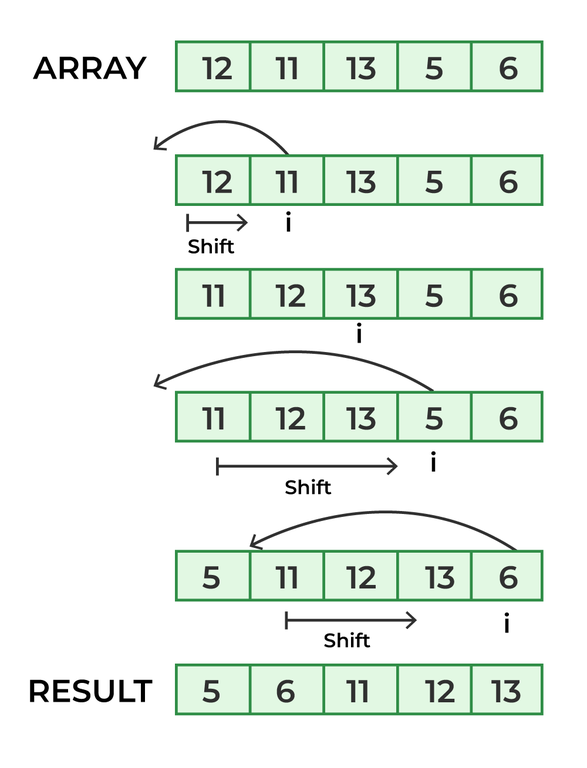Related Articles

# Java Program for Insertion Sort

Insertion sort is a simple sorting algorithm that works the way we sort playing cards in our hands. In this article, we will write the program on Insertion Sort in Java.## Algorithm of Insertion Sort

The algorithm of Insertion Sort is mentioned below:

1. Variable declared i=1
2. Traverse the Array till i<N
• If arr[i]<arr[i-1] then arr[j]=value present after shifting the elements of the array from j to i-1.
3. Return the Sorted Array.

## Java

 `// Java program for implementation of Insertion Sort ` `class` `InsertionSort { ` `    ``// Function to sort array using insertion sort ` `    ``void` `sort(``int` `arr[]) ` `    ``{ ` `        ``int` `n = arr.length; ` `        ``for` `(``int` `i = ``1``; i < n; ++i) { ` `            ``int` `key = arr[i]; ` `            ``int` `j = i - ``1``; ` ` `  `            ``// Move elements of arr[0..i-1], that are ` `            ``// greater than key, to one position ahead ` `            ``// of their current position ` `            ``while` `(j >= ``0` `&& arr[j] > key) { ` `                ``arr[j + ``1``] = arr[j]; ` `                ``j = j - ``1``; ` `            ``} ` `            ``arr[j + ``1``] = key; ` `        ``} ` `    ``} ` ` `  `    ``// A utility function to print array of size n ` `    ``static` `void` `printArray(``int` `arr[]) ` `    ``{ ` `        ``int` `n = arr.length; ` `        ``for` `(``int` `i = ``0``; i < n; ++i) ` `            ``System.out.print(arr[i] + ``" "``); ` ` `  `        ``System.out.println(); ` `    ``} ` ` `  `    ``// Driver method ` `    ``public` `static` `void` `main(String args[]) ` `    ``{ ` `        ``int` `arr[] = { ``12``, ``11``, ``13``, ``5``, ``6` `}; ` ` `  `        ``InsertionSort ob = ``new` `InsertionSort(); ` `        ``ob.sort(arr); ` ` `  `        ``printArray(arr); ` `    ``} ` `}`

Output

```5 6 11 12 13
```

#### The complexity of the above method

Time Complexity: O(N^2)
Auxiliary Space: O(1)

## Reference

Please refer complete article on Insertion Sort for more details!

Whether you're preparing for your first job interview or aiming to upskill in this ever-evolving tech landscape, GeeksforGeeks Courses are your key to success. We provide top-quality content at affordable prices, all geared towards accelerating your growth in a time-bound manner. Join the millions we've already empowered, and we're here to do the same for you. Don't miss out - check it out now!

Previous
Next